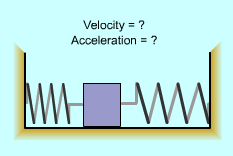Ch 3. Derivatives II Multimedia Engineering Math Higher OrderDerivatives RelatedRates Differential Newton'sMethod
 Chapter 1. Limits 2. Derivatives I 3. Derivatives II 4. Mean Value 5. Curve Sketching 6. Integrals 7. Inverse Functions 8. Integration Tech. 9. Integrate App. 10. Parametric Eqs. 11. Polar Coord. 12. Series Appendix Basic Math Units Search eBooks Dynamics Fluids Math Mechanics Statics Thermodynamics Author(s): Hengzhong Wen Chean Chin Ngo Meirong Huang Kurt Gramoll ©Kurt GramollMATHEMATICS - CASE STUDY IntroductionSpring-mounted Slider Chuck built a spring-mounted slider to illustrate simple harmonic motion to his son . He pushes the slider to the left side, releases it and the slider starts to move in the horizontal guide. He is curious about the velocity and acceleration 2 second after the slider starts moving. What is known: The displacement of the slider is expressed by s = 20 sin5t. The time, t, is 2 seconds Questions What is the velocity and acceleration of the slider 2 seconds after it starts to move? Approach The first order derivative of the displacement with respect to time is velocity, ds/dt = v. The second order derivative of the displacement with respect to time is acceleration, d2s/dt2 = a. The first order derivative of velocity with respect to time is acceleration, dv/dt = a.